# T1, T2, and T2*

T1, T2, and T2*

Introduction

We have already introduced relaxation times T1, T2, and T2*. In this chapter, we discuss the physical properties behind them and see what conditions cause them to be increased or decreased. As we have discussed before, T1 and T2 are inherent properties of tissues and are thus fixed for a specific tissue (at a given magnetic field strength). The parameter T2*, however, also depends on inhomogeneities in the main magnetic field, but again is fixed for a specific tissue within a given external magnetic environment.

T1 Relaxation Time

The term relaxation means that the spins are relaxing back into their lowest energy state or back to the equilibrium state. (Equilibrium by definition is the lowest energy state possible.) Once the radio frequency (RF) pulse is turned off, the protons will have to realign with the axis of the B0 magnetic field and give up all their excess energy.

T1 is called the longitudinal relaxation time because it refers to the time it takes for the spins to realign along the longitudinal (z)-axis. T1 is also called the spin-lattice relaxation time because it refers to the time it takes for the spins to give the energy they obtained from the RF pulse back to the surrounding lattice in order to go back to their equilibrium state.

Immediately after the 90° pulse, the magnetization Mxy precesses within the x-y plane, oscillating around the z-axis with all protons rotating in phase (Fig. 4-1). After the magnetization has been flipped 90° into the x-y plane, the RF pulse is turned off. A general principle of thermodynamics is that every system seeks its lowest energy level. Therefore, after the RF pulse is turned off, two things will occur:

• The spins will go back to the lowest energy state.

• The spins will get out of phase with each other.

These events result from two simultaneous but separate processes occurring after the RF pulse is turned off (Fig. 4-2):

• The Mxy component of the magnetization vector decreases rapidly.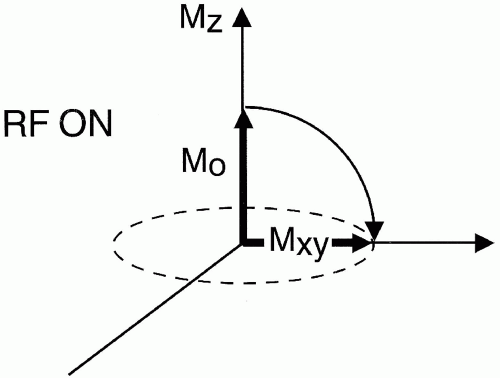Figure 4-1. After the RF pulse, the longitudinal magnetization vector is flipped into the x-y plane.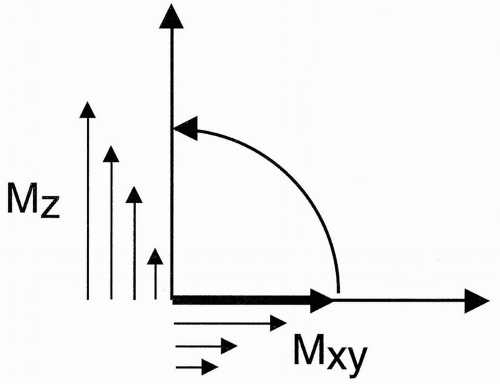Figure 4-2. Once the RF is turned off, the transverse magnetization vector begins to decay while the longitudinal component begins to recover.

• The Mz component slowly recovers along the z-axis.

Question: What time constant characterizes the rate at which the Mz component recovers its initial magnetization M0?

When we first discussed magnetization, we said that the protons start to line up with the external magnetic field at a rate given by T1. (Because T1 is a time [in sec], the rate is 1/T1 [in sec-1].) The same phenomenon occurs when we flip the magnetization M0 away from the longitudinal z-axis and then allow it to realign with the main magnetic field after an RF pulse. The rate at which Mz recovers to M0 is also given by T1.

Immediately after a 90° pulse, all magnetization is in the x-y plane. The Mz component then starts to grow at a rate characterized by T1 (Fig. 4-3):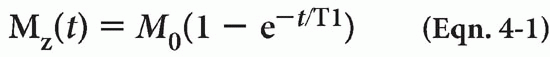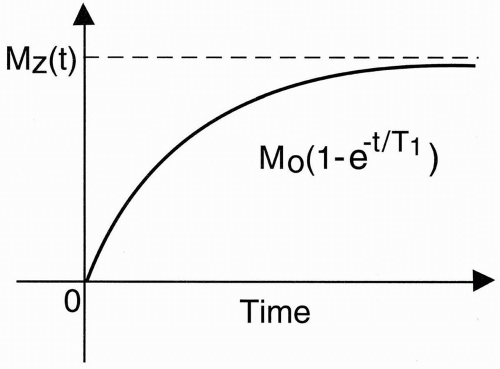Figure 4-3. The graph of recovery of longitudinal magnetization with the growth rate of T1.

T2 Relaxation Time

Figure 4-2 also shows rapid decay of the Mxy component after the RF pulse is turned off.

Question: What time constant characterizes the rate at which the Mxy component decays?

As the longitudinal magnetization vector Mz recovers, the transverse vector Mxy decays at a rate characterized by T2 (Fig. 4-4):Realize that the recovery of magnetization along the z-axis and the decay of magnetization within the x-y plane are two independent processes occurring at two different rates (Fig. 4-5). Take a simple exponential process. We would expect the rate at which this process decays in the x-y plane to be the same as that at which it grows along the z-axis (Fig. 4-6). This is not the same in the MR system we are discussing because this system involves a much more complicated process. T2 decay occurs 5 to 10 times more rapidly than T1 recovery (Fig. 4-7). To understand this, we need to understand the concept of dephasing.

Dephasing. After the 90° RF pulse is turned off, all spins are in phase; they are all lined up in the same direction and spinning at the same frequency ω0. There are two phenomena that will make the spins get out of phase: interactions between spins and external field inhomogeneities.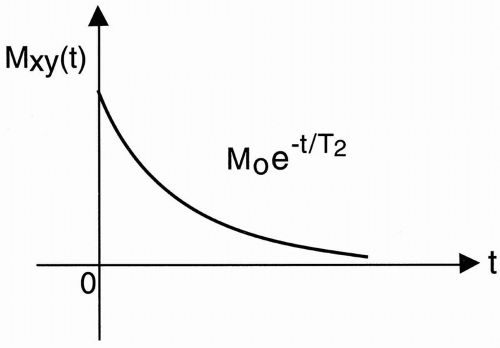Figure 4-4. The graph of transverse magnetization with the decay rate of T2 and RF off.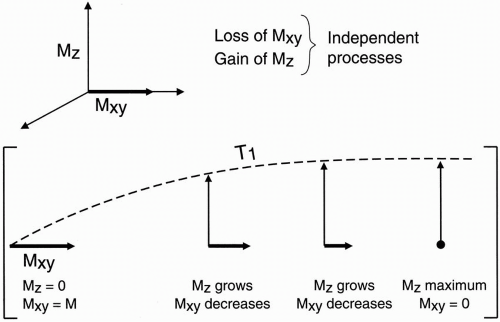Figure 4-5. The recovery of longitudinal magnetization and the decay of transverse magnetization occur at the same time but are independent of each other with the RF off.

1. Interactions Between Individual Spins

When two spins are next to each other, the magnetic field of one proton affects the proton next to it. Assume one proton is aligned with the field and the other is against it (Fig. 4-8). The proton aligned with B0 creates a slightly higher magnetic field for its neighbor so that proton #1 is exposed to the magnetic field B0 plus a small magnetic field created by the other proton (δB). The precessional frequency of the proton then will increase slightly as ω (proton #1) = (B0 + δB)

On the other side, the other proton is exposed to a slightly weaker magnetic field because the other proton points against B0. Therefore, its overall magnetic field strength exposure will be slightly less. The precessional frequency of proton #2 then will decrease slightly as

ω (proton #2) = (B0 − δB)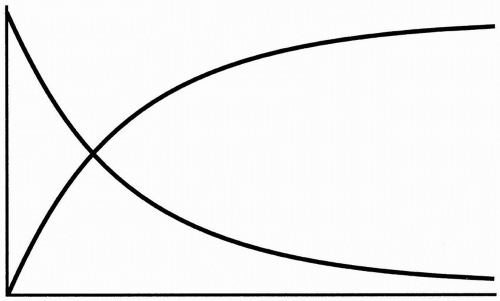Figure 4-6. The rate of growth and decay of a simple exponential process is expected to be similar.

The difference in the magnetic environment created by these proton-proton interactions may be very small, but it makes a difference in the overall homogeneity of the magnetic field to which the spins are exposed. Thus, the first cause of dephasing is inherent in the tissue. It is called a spin-spin interaction.